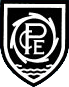# Learning  »  Class Pages  »  Year 6  »  Class Work  »  Maths Work

Y6 Autumn 1

We are focusing on number and place value and the four operations.

### Y6 Curriculum: Number - number and place value

• read, write, order and compare numbers up to 10,000,000 and determine the value of each digit
• round any whole number to a required degree of accuracy
• use negative numbers in context, and calculate intervals across 0
• solve number and practical problems that involve all of the above

### Y6 Curriculum: Number - addition, subtraction, multiplication and division

• multiply multi-digit numbers up to 4 digits by a two-digit whole number using the formal written method of long multiplication
• divide numbers up to 4 digits by a two-digit whole number using the formal written method of long division, and interpret remainders as whole number remainders, fractions, or by rounding, as appropriate for the context
• divide numbers up to 4 digits by a two-digit number using the formal written method of short division where appropriate, interpreting remainders according to the context
• perform mental calculations, including with mixed operations and large numbers
• identify common factors, common multiples and prime numbers
• use their knowledge of the order of operations to carry out calculations involving the 4 operations
• solve addition and subtraction multi-step problems in contexts, deciding which operations and methods to use and why
• solve problems involving addition, subtraction, multiplication and division
• use estimation to check answers to calculations and determine, in the context of a problem, an appropriate degree of accuracy
Pool-in-Wharfedale CE Primary School Arthington Lane, Pool-in-Wharfedale, Otley, LS21 1LG